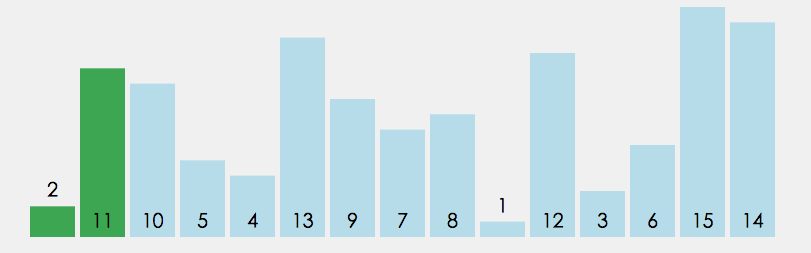﻿ Java深入了解数据结构中常见的排序算法_java_脚本之家
java# Java深入了解数据结构中常见的排序算法

## 一，概念

### 2，稳定性## 二，排序详解### 1，插入排序

#### ①直接插入排序

1. 有序区间

2. 无序区间``` public static void main(String[] args) {

int[] array = {12,5,9,34,6,8,33,56,89,0,7,4,22,55,77};
insertSort(array);
System.out.println(Arrays.toString(array));
}```
```  /**
* 时间复杂度：
*        最好：O(N)   -> 数据是有序的
*        最坏：O(N^2) -> 无序的数据
* 空间复杂度：O(1)
* 稳定性：稳定排序
* @param array
*/
public static void insertSort(int[] array) {
for(int i = 1;i < array.length;i++) {//n-1
int tmp = array[i];
int j = i-1;
for(; j >= 0;j--) {//n-1
if(array[j] > tmp) {
array[j+1] = array[j];
}else{
//array[j+1] = tmp;
break;
}
}
array[j+1] = tmp;
}
}```②希尔排序

1. 希尔排序是对直接插入排序的优化。

2. 当gap > 1时都是预排序，目的是让数组更接近于有序。当gap == 1时，数组已经接近有序的了，这样就会很 快。这样整体而言，可以达到优化的效果。我们实现后可以进行性能测试的对比。```   /**
* 时间复杂度：不好算  n^1.3 - n^1.5 之间
* 空间复杂度：O(1)
* 稳定性：不稳定的排序
*      技巧：如果在比较的过程当中 没有发生跳跃式的交换 那么就是稳定的
* @param array
*
*
* @param array 排序的数组
* @param gap   每组的间隔  -》 组数
*/
public static void shell(int[] array,int gap) {
for (int i = gap; i < array.length; i++) {
int tmp = array[i];
int j = i-gap;
for (; j >= 0; j -= gap) {
if(array[j] > tmp) {
array[j+gap] = array[j];
}else {
break;
}
}
array[j+gap] = tmp;
}
}```
```public static void main(String[] args) {

int[] array = {12,5,9,34,6,8,33,56,89,0,7,4,22,55,77};
shell(array,5);
System.out.println(Arrays.toString(array));
}```### 2，选择排序

#### ①直接选择排序```public static void main(String[] args) {

int[] array = {12,5,9,34,6,8,33,56,89,0,7,4,22,55,77};
selectSort(array);
System.out.println(Arrays.toString(array));
}```
```  /**
* 时间复杂度：
*      最好：O(N^2)
*      最坏：O(N^2)
* 空间复杂度：O(1)
* 稳定性：不稳定的
* @param array
*/
public static void selectSort(int[] array) {
for (int i = 0; i < array.length; i++) {
for (int j = i+1; j < array.length; j++) {
if(array[j] < array[i]) {
int tmp = array[i];
array[i] = array[j];
array[j] = tmp;
}
}
}
}```#### ②堆排序```  public static void main(String[] args) {

int[] array = {12,5,9,34,6,8,33,56,89,0,7,4,22,55,77};
heapSort(array);
System.out.println(Arrays.toString(array));
}```
```   public static void siftDown(int[] array,int root,int len) {
int parent = root;
int child = 2*parent+1;
while (child < len) {
if(child+1 < len && array[child] < array[child+1]) {
child++;
}
//child的下标就是左右孩子的最大值下标
if(array[child] > array[parent]) {
int tmp = array[child];
array[child] = array[parent];
array[parent] = tmp;
parent = child;
child = 2*parent+1;
}else {
break;
}
}
}

public static void createHeap(int[] array) {
//从小到大排序 -》 大根堆
for (int i = (array.length-1 - 1) / 2;  i >= 0 ; i--) {
siftDown(array,i,array.length);
}
}

/**
* 时间复杂度：O(N*logN)  都是这个时间复杂度
* 复杂度：O(1)
* 稳定性：不稳定的排序
* @param array
*/
public static void heapSort(int[] array) {
createHeap(array);//O(n)
int end = array.length-1;
while (end > 0) {//O(N*logN)
int tmp = array[end];
array[end] = array;
array = tmp;
siftDown(array,0,end);
end--;
}
}```### 3，交换排序

#### ①冒泡排序``` public static void main(String[] args) {

int[] array = {12,5,9,34,6,8,33,56,89,0,7,4,22,55,77};
bubbleSort(array);
System.out.println(Arrays.toString(array));
}```
```   /**
* 时间复杂度：
*         最好最坏都是O(n^2)
* 空间复杂度：O(1)
* 稳定性：稳定的排序
*      冒泡  直接插入
* @param array
*/
public static void bubbleSort(int[] array) {
for (int i = 0; i < array.length-1; i++) {
for (int j = 0; j < array.length-1-i; j++) {
if(array[j] > array[j+1]) {
int tmp = array[j];
array[j] = array[j+1];
array[j+1] = tmp;

}
}
}
}```#### ②快速排序

1. 从待排序区间选择一个数，作为基准值(pivot)；

2. Partition: 遍历整个待排序区间，将比基准值小的（可以包含相等的）放到基准值的左边，将比基准值大的（可 以包含相等的）放到基准值的右边；

3. 采用分治思想，对左右两个小区间按照同样的方式处理，直到小区间的长度 == 1，代表已经有序，或者小区间 的长度 == 0，代表没有数据。```public static void main(String[] args) {

int[] array = {12,5,9,34,6,8,33,56,89,0,7,4,22,55,77};
quickSort1(array);
System.out.println(Arrays.toString(array));
}```
```public static int partition(int[] array,int low,int high) {
int tmp = array[low];
while (low < high) {
while (low < high && array[high] >= tmp) {
high--;
}
array[low] = array[high];
while (low < high && array[low] <= tmp) {
low++;
}
array[high] = array[low];
}
array[low] = tmp;
return low;
}
public static void quick(int[] array,int start,int end) {
if(start >= end) {
return;
}
int mid = (start+end)/2;
int pivot = partition(array,start,end);
quick(array,start,pivot-1);
quick(array,pivot+1,end);
}

/**
* 时间复杂度：
*         最好：O(n*logn)  均匀的分割下
*         最坏：o(n^2)     数据有序的时候
* 空间复杂度：
*        最好：logn
*        最坏：O(n)
* 稳定性：不稳定的排序
*
* k*n*logn
* 2
* 1.2
* @param array
*/
public static void quickSort1(int[] array) {
quick(array,0,array.length-1);
}```### 4，归并排序``` public static void main(String[] args) {

int[] array = {12,5,9,34,6,8,33,56,89,0,7,4,22,55,77};
mergeSort1(array);
System.out.println(Arrays.toString(array));
}```
```public static void merge(int[] array,int low,int mid,int high) {
int s1 = low;
int e1 = mid;
int s2 = mid+1;
int e2 = high;
int[] tmp = new int[high-low+1];
int k = 0;//代表tmp数组的下标
while (s1 <= e1 && s2 <= e2) {
if(array[s1] <= array[s2]) {
tmp[k++] = array[s1++];
}else {
tmp[k++] = array[s2++];
}
}

//有2种情况
while (s1 <= e1){
//说明第2个归并段没有了数据 把第1个归并段剩下的数据 全部拷贝过来
tmp[k++] = array[s1++];
}

while (s2 <= e2) {
//说明第1个归并段没有了数据 把第2个归并段剩下的数据 全部拷贝过来
tmp[k++] = array[s2++];
}
//tmp数组当中 存储的就是当前归并好的数据

for (int i = 0; i < tmp.length; i++) {
array[i+low] = tmp[i];
}
}
public static void mergeSortInternal(int[] array,int low,int high) {
if(low >= high) {
return;
}
int mid = (low+high) / 2;
mergeSortInternal(array,low,mid);
mergeSortInternal(array,mid+1,high);
//合并的过程
merge(array,low,mid,high);
}

/**
* 时间复杂度： O(N*log n)
* 空间复杂度：O(N)
* 稳定性：稳定的
* @param array
*/
public static void mergeSort1(int[] array) {
mergeSortInternal(array, 0,array.length-1);
}```